# Texas Go Math Grade 8 Lesson 10.2 Answer Key Surface Area of Cylinders

Refer to our Texas Go Math Grade 8 Answer Key Pdf to score good marks in the exams. Test yourself by practicing the problems from Texas Go Math Grade 8 Lesson 10.2 Answer Key Surface Area of Cylinders.

## Texas Go Math Grade 8 Lesson 10.2 Answer Key Surface Area of Cylinders

Modeling the Surface Area of a Cylinder

Just as you did with a prism, you can use a net to help you find a formula for the surface area of a cylinder.The lateral area of a cylinder is the area of the curved surface that connects the two bases. The net shows that the lateral surface is a rectangle.
Use the diagram of the oatmeal container and its net.
A. Imagine unrolling the container’s lateral surface to form a rectangle
What dimension of the cylinder matches the rectangle’s length? ____________
What dimension of the cylinder matches the rectangle’s height? ____________
What dimension of the cylinder matches the rectangle’s length? 2πr
What dimension of the cylinder matches the rectangle’s height? h
B. Express the lateral area of a cylinder in words and as a formula using the given variables.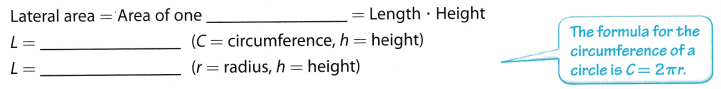C. Express the total surface area of a cylinder in words and as a formula using the given variables.Reflect

Question 1.
Communicate Mathematical Ideas How is the process for finding lateral and total surface area of a cylinder-like the process for a prism?
For both prisms, you can draw a net to help you find the areas of the faces. Add the areas together to find the surface area. The lateral area of a cylinder is the area of the curved surface that connects the two bases. The net shows that the lateral surface is a rectangle. The total surface area S of a cylinder with height h and radius r is twice the area of a base B plus the lateral area.

Find the lateral and total surface area of each cylinder. Round your answers to the nearest tenth. Use 3.14 for π.

Question 2.Given,
r = 6 cm
h = 13 cm
We know that,
Lateral Surface Area of a Cylinder = 2πrh
π = 3.14
LSA = 2 × 3.14 × 6 × 13
LSA = 489.84
489.84 to the nearest tenth is 489.8 sq. cm
Total Surface Area = 2πr² + L
TSA = 2 × 3.14 × 6 × 6 + 489.8
TSA = 226.08 + 489.8 = 715.88
715.88 to the nearest tenth is 715.9

Question 3.Given,
d = 7 ft
r = 7/2 = 3.5 ft
h = 5 ft
We know that,
Lateral Surface Area of a Cylinder = 2πrh
π = 3.14
LSA = 2 × 3.14 × 3.5 × 5
LSA = 109.9 sq. ft
109.9 to the nearest tenth is 110.
Total Surface Area = 2πr² + L
TSA = 2 × 3.14 × 3.5 × 3.5 + 109.9
TSA = 76.93 + 109.9 = 186.83 sq. ft
186.83 to the nearest tenth is 186.8

Question 4.
How many square inches of cardboard are needed for the lateral area of the raisin container shown? What is the total surface area of the container? Round your answers to the nearest tenth. Use 3.14 for π.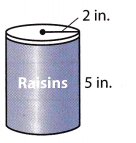Given,
r = 2 in
h = 5 in.
We know that,
Lateral Surface Area of a Cylinder = 2πrh
π = 3.14
LSA = 2 × 3.14 × 2 × 5
LSA = 62.8 sq. in.
62.8 to the nearest tenth is 62.8.
Total Surface Area = 2πr² + L
TSA = 2 × 3.14 × 2 × 2 + 62.8
TSA = 25.12 + 62.8 = 87.92 sq. in
87.92 to the nearest tenth is 87.9 sq. in.

Question 1.
Draw a net of the cylinder. Label the radius and the height. Then find the lateral area and total surface area. Round your answers to the nearest tenth. Use 3.14 for π. (Explore Activity and Example 1)Find the circumference of the circle. Use 3.14 for π.
C = 2πr ≈ 2 ∙ 3.14 ∙ ________ ≈ ________ inches
The lateral area is the circumference times the height of the can.
L ≈ 31.4 ∙ _______ ≈ ______ in2
Find the area of the two bases. Use 3.14 for π.
2B = 2πr2 ≈ (3.14) (_________ ) ≈ _______ in2
Add the area of the bases and the lateral area.
S ≈ ________ + __________ ≈ __________ in2
Find the circumference of the circle. Use 3.14 for π.
C = 2πr ≈ 2 ∙ 3.14 ∙ 5 ≈ 31.4 inches
The lateral area is the circumference times the height of the can.
L ≈ 31.4 ∙ 8 ≈ 251.2 in2
Find the area of the two bases. Use 3.14 for π.
2B = 2πr2 ≈ 2 (3.14) (25) ≈ 157 in2
Add the area of the bases and the lateral area.
S ≈ 157 + 251.2 ≈ 408 in2

Question 2.
A can of tuna fish has a height of 1 inch and a diameter of 3 inches. How many square inches of paper are needed for the label? How many square inches of metal are needed to make the can including the top and bottom? Round your answers to the nearest whole number. Use 3.14 for π. (Example 2)It takes about __________ square inches of paper to make the label.
It takes about ___________ square inches of metal to make the can.
A can of tuna fish has a height of 1 inch and a diameter of 3 inches.
h = 1 in.
d = 3 in
r = d/2 = 3/2 = 1.5 in.
We know that,
Lateral Surface Area of a Cylinder = 2πrh
π = 3.14
LSA = 2 × 3.14 × 1.5 × 1
LSA = 9.42 sq. in.
Total Surface Area = 2πr² + L
TSA = 2 × 3.14 × 1.5 × 1.5 + 9.42 = 23.55 sq. in.
It takes about 9.42 sq. in. square inches of paper to make the label.
It takes about 23.55 sq. in. square inches of metal to make the can.

Essential Question Check-In

Question 3.
How do you find the total surface area of a cylinder?
The surface area of a cylinder is the area occupied by its surface in a three-dimensional space.
The Surface Area of Cylinder = Curved Surface + Area of Circular bases
S.A. = 2πr (h + r) sq.unit
Where, π (Pi) = 3.142 or = 22/7
r = Radius of the cylinder
h = Height of the cylinder
TSA = 2π × r × h + 2πr²= 2πr (h + r) Square units

Find the lateral and total surface area of each cylinder. Round your answers to the nearest tenth. Use 3.14 for π.

Question 4.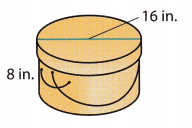h = 8 in.
d = 16 in
r = d/2 = 16/2 = 8 in.
We know that,
Lateral Surface Area of a Cylinder = 2πrh
π = 3.14
LSA = 2 × 3.14 × 8 × 8
LSA = 401.92 sq. in.
Total Surface Area = 2πr² + L
TSA = 2 × 3.14 × 8 × 8 + 401.92 = 401.92 + 401.92 = 803.84
803.84 to the nearest tenth is 803.8 sq. in

Question 5.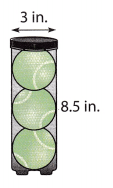h = 8.5 in.
d = 3 in
r = d/2 = 3/2 = 1.5 in.
We know that,
Lateral Surface Area of a Cylinder = 2πrh
π = 3.14
LSA = 2 × 3.14 × 1.5 × 8.5
LSA = 80.07 sq. in.
Total Surface Area = 2πr² + L
TSA = 2 × 3.14 × 1.5 × 1.5 + 80.07 = 14.13 + 80.07 = 94.2 sq. in.

Question 6.Given,
r = 6 cm
h = 9 cm
We know that,
Lateral Surface Area of a Cylinder = 2πrh
π = 3.14
LSA = 2 × 3.14 × 6 × 9
LSA = 339.12 sq. cm
339.12 to the nearest tenth is 339.1 sq. cm
Total Surface Area = 2πr² + L
TSA = 2 × 3.14 × 6 × 6 + 339.12
TSA = 226.08 + 339.12 = 565.2 sq. cm
565.2 to the nearest tenth is 565.2 sq. cm.

Question 7.
A container of bread crumbs has a radius of 4 inches and a height of 8 inches.
Given,
A container of bread crumbs has a radius of 4 inches and a height of 8 inches.
r = 4 in
h = 8 in.
We know that,
Lateral Surface Area of a Cylinder = 2πrh
π = 3.14
LSA = 2 × 3.14 × 4 × 8
LSA = 200.96 sq. in.
200.96 to the nearest tenth is 201 sq. in.
Total Surface Area = 2πr² + L
TSA = 2 × 3.14 × 4 × 4 + 201
TSA = 100.48 + 201 = 301.48 sq. in.

Question 8.
A rain barrel has a diameter of 18 inches and a height of 3 feet.
Given,
A rain barrel has a diameter of 18 inches and a height of 3 feet.
h = 3 ft
d = 18 ft
r = d/2 = 18/2 = 9 ft
We know that,
Lateral Surface Area of a Cylinder = 2πrh
π = 3.14
LSA = 2 × 3.14 × 9 × 3
LSA = 169.56 sq. ft.
Total Surface Area = 2πr² + L
TSA = 2 × 3.14 × 9 × 9 + 80.07 = 508.68 + 169.56 = 678.24 sq. ft

Question 9.
A drum has a diameter of 14 inches and a height of 5.5 inches.
Given,
A drum has a diameter of 14 inches and a height of 5.5 inches.
h = 5.5 in.
d = 14 in.
r = d/2 = 14/2 = 7 in.
We know that,
Lateral Surface Area of a Cylinder = 2πrh
π = 3.14
LSA = 2 × 3.14 × 7 × 5.5
LSA = 241.78 sq. in.
Total Surface Area = 2πr² + L
TSA = 2 × 3.14 × 7 × 7 + 241.78 = 307.72 + 241.78 = 549.5 sq. in.

Question 10.
Multistep A pipe is 25 inches long and has a diameter of 5 inches. What is the lateral area of the pipe to the nearest tenth? Use 3.14 for π.
Given,
A pipe is 25 inches long and has a diameter of 5 inches
The pipe is in the shape of a cylinder.
Height = h = 25 inches
Diameter = D = 5 inches.
Radius = R = D/2 = 5/2. = 2.5 inches.
The formula for the lateral surface area of the cylinder =2πrh
π = 3.14
= 2 x 3.14 x 2.5 x 25.
= 392.5 sq. inches.
392.5 nearest to the tenth is 392 sq. inches.

Question 11.
Multistep A size D battery has a diameter of 32 millimeters and a length of 5.6 centimeters. What is the lateral area of the battery to the nearest square centimeter? Use 3.14 for π.
Given,
A size D battery has a diameter of 32 millimeters and a length of 5.6 centimeters.
32 millimeters = 32/10 = 3.2 centimeters
The formula for the lateral surface area of the cylinder =2πrh
d = r/2
d = 3.2/2 = 1.6 cm
LSA = 2 × 3.14 × 1.6 × 5.6 = 56.2 sq. cm

Question 12.
What If? Carol is designing an oatmeal container. Her first design is a rectangular prism with a height of 12 inches, a width of 8 inches, and a depth of 3 inches.
a. What is the total surface area of the container to the nearest square inch? Use 3.14 for π.
The formula for the total surface area of the rectangular prism = 2(lb + bh + lh).
Length = 3 inches
Breath = 8 inches.
Height = 12 inches
= 2(3 x 8 + 8 x 12 + 3 x 12)
= 2(24 + 96 + 36)
= 312 sq. inches.
The total surface area of the rectangular prism = 312 sq. inches.

b. Carol wants to redesign the package as a cylinder with the same total surface area as the prism in part a. If the radius of the cylinder is 2 inches, what is the height of the cylinder? Round your answer to the nearest inch. Use 3.14 for π.
The formula for the total surface cylinder = 2πr² + 2πrh.
The total surface area of the rectangular prism = 312 cu. inches.
2(3.12)(4) + 2 (3.14)(2)h = 312
24.96 + 6.28h = 312
6.2h = 312 + 24.96
6.2h = 336.96
h = 54.34.
The height of the cylinder = 54.34 inches

Question 13.
Vocabulary How do the lateral and total surface area of a cylinder differ?
The lateral surface area means the curved surface area.
The total surface area means the curved surface area plus the surface area of the bottom and the top.

H.O.T. Focus on Higher Order Thinking

Question 14.
Multiple Representations The formula for the total surface area of a cylinder is S = 2πr2 + 2πrh. Explain how you could use the Distributive Property to write the formula another way.
Distribute property = ab + ac = a(b + c).
The formula for the total surface cylinder = 2πr² + 2πrh.
2πr² + 2πrh = 2r(πr + πh).
The formula of the total surface area of the cylinder in another way = 2r(πr + πh).

Question 15.
Persevere in Problem Solving The treasure chest shown is a composite figure. What is the surface area of the treasure chest to the nearest square foot? Explain how you found your answer.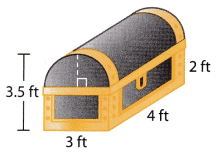The treasure chest is rectangular.
The total surface area of the rectangular prism for composite figures = 2Bh + Ph.
Perimeter of a rectangle = 2(l + w)
Length = 3 feets.
Width = 4 feets.
Height = 2 feets.
= 2(3 + 4).
= 14 feets.
Find base of the rectangle = ½ bh.
b = 3 feets.
h = 3.5 feets.
B = ½ x 3 x 3.5
B = 5.25 sq. feets.
The total surface area of the rectangular prism for composite figures = 2(5.25)(2) + (14)(2).
= 21 + 28
= 49 sq. feets.

Question 16.
Analyze Relationships A square prism has the same height as the cylinder shown. The perimeter of the prism’s base equals the circumference of the cylinder’s base.a. Find the side length of the prism’s base to the nearest centimeter. ___________
The formula for the base area of the cylinder = πr²
Height = 12 cm
= π x 7².
= 153.86 sq. cm.
The base area of the cylinder = 153.86 sq. cm.

b. Which figure has the greater lateral area? Which has the greater total surface area? Which has the greater volume? Explain.

Question 17.
Communicate Mathematical Ideas A cylinder has a circumference of 16π inches and its height is half the radius of the cylinder. What is the total surface area of the cylinder? Give your answer in terms of π. Explain how you found your answer.
Given that,
The circumference of the cylinder = 16π inches.
The height of the cylinder is half of the radius.
The formula for the radius of the cylinder = 2πr
2πr = 16π
r = 16π/2π
r = 8